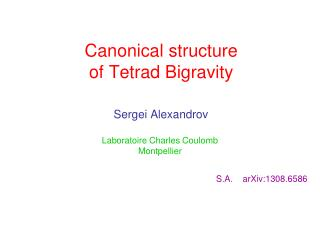# Canonical structure of Tetrad Bigravity - PowerPoint PPT PresentationDownload PresentationDownload Presentation## Canonical structure of Tetrad Bigravity

- - - - - - - - - - - - - - - - - - - - - - - - - - - E N D - - - - - - - - - - - - - - - - - - - - - - - - - - -
##### Presentation Transcript

1. Canonical structure of Tetrad Bigravity Laboratoire Charles Coulomb Montpellier Sergei Alexandrov S.A. arXiv:1308.6586

2. Plan of the talk • Introduction: massiveandbimetricgravitty in the metric and tetrad formulations • Canonical structure of GR in the Hilbert-Palatini formulation • Canonical structure of tetrad bigravity • Conclusions

3. (In vacuum) is transverse and traceless and carries 5 d.o.f. At the non-linear level ― Einstein-Hilbert action plus a potential an extra fixed metric • has the flat space as a solution • reduces to for • and Massive gravity The idea: to give to the graviton a non-vanishing mass At the linearized level ― Fierz-Pauli theory breaks gauge invariance Diffeomorphism symmetry is broken

4. Bimetric gravity Two dynamical metrics coupled by a non-derivative interaction term • Diffeomorphism symmetry: • diagonal― preserved by the mass term • off-diagonal ― broken by the mass term The theory describes one massless and one massive gravitons In fact, not only…

5. The trace becomes dynamical and describes a scalar ghost― the Hamiltonian is unbounded from below In the canonical language: The lapse and shift do not enter linearly and therefore are not Lagrange multipliers anymore (In the FP Lagrangian generates a second class constraint removing one d.o.f.) Boulware-Deser ghost Massive gravity describes a theory with 6 d.o.f. Boulware,Deser ’72 : Is it possible to find an interaction potential which is free from the ghost pathology?

6. Ghost-free potentials There is a three-parameter family of ghost-free potentials deRham,Gabadadze,Tolley ’10 : Symmetric polynomials: The ghost is absent because the lapse appears again as Lagrange multiplier and generates a (second class) constraint Hassan,Rosen ’11 How to deal with the awkward square root structure?

7. = Symmetricity condition = The mass term in the tetrad formalism (in 4d): • The symmetricity constraint follows from e.o.m. • The model should be absent from the BD ghost antisymmetricity of the wedge product linear in linear in Hinterbichler,Rosen ’12 Tetrad reformulation The idea: to reformulate the theory using tetrads The important property:

8. Cartan equations Bimetric gravity in the tetrad formulation The model The Hilbert-Palatini action: The mass term:

9. the phase space the primary constraints Hilbert-Palatini action 3+1 decomposition:

10. The kinetic term: where primary constraints secondary constraints Non-covariant description Solve constraints explicitly

11. We also need Covariant description Don’t solve constraints explicitly secondary constraints The symplectic structure is given byDirac brackets

12. does not depend on Covariant description Non-covariant description Phase space: Phase space: + s.c. constraints Symplectic structure: Symplectic structure: Dirac brackets canonical Poisson brackets + constraints affected by the mass term Tetrad bigravity: phase space The second class constraints of the two HP actions are not affected by the mass term

13. not expressible in terms of The total set of primary constraints: diagonal sector off-diagonal sector weakly commute with all primary constraints It remains to analyze the stability of Tetrad bigravity: primary constraints Decomposition of the mass term: where

14. secondary constraint condition on Lagrange multipliers Symmetricity condition Tetrad bigravity: symmetricity condition Stabilization of : Crucial property: where mixed metric Tetrad and metric formulations are indeed equivalent on-shell

15. fixing Lagrange multipliers secondary constraint are second class Tetrad bigravity: constraint algebra

16. Stability condition for are second class fixes or secondary constraint stability condition for Tetrad bigravity: secondary constraint One can compute explicitly where

17. 14 dim. phase space space or 7 degrees of freedom 2 ― massless graviton 5 ― massive graviton Summary of the phase space structure • Phase space (in non-covariant description): + 2×(9+9+3+3) = 48 • Second class constraints: – (6+3+3+1+1) = –14 • First class constraints: – 2×(6+3+1) = –20 The BD ghost is absent!

18. Open problems • Superluminality, instabilities, tachyonic modes… • Partially massless theory • Degenerate sectors Additional gauge symmetry reducing the number of d.o.f. of the massive graviton from 5 to 4. Is it possible? What happens with the theory for configurations where some of the invertibility properties fail? Detailed study of the stability condition for